# How to Calculate and Solve for Mean Bubble Point in a Fluid | The Calculator Encyclopedia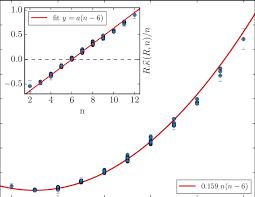The image above represents mean bubble point.

To compute for the mean bubble point, one essential parameter is needed and the parameter is Glass gas solubility parameter (x).

The formula for calculating mean bubble point:

Pb* = 10x

Where:

Pb* = Mean Bubble Point
x = Glass Gas Solubility Parameter

Let’s solve an example;
Given that the glass gas solubility parameter is 12. Find the mean bubble point?

This implies that;

x = Glass Gas Solubility Parameter = 12

Pb* = 10x
Pb* = 1012
Pb* = 1000000000000

Therefore, the Mean bubble point is 1000000000000.

Nickzom Calculator – The Calculator Encyclopedia is capable of calculating the mean bubble point.

To get the answer and workings of the mean bubble point using the Nickzom Calculator – The Calculator Encyclopedia. First, you need to obtain the app.

You can get this app via any of these means:

To get access to the professional version via web, you need to register and subscribe for NGN 1,500 per annum to have utter access to all functionalities.
You can also try the demo version via https://www.nickzom.org/calculator

Apple (Paid) – https://itunes.apple.com/us/app/nickzom-calculator/id1331162702?mt=8
Once, you have obtained the calculator encyclopedia app, proceed to the Calculator Map, then click on Petroleum under EngineeringNow, Click on Fluid Properties under Petroleum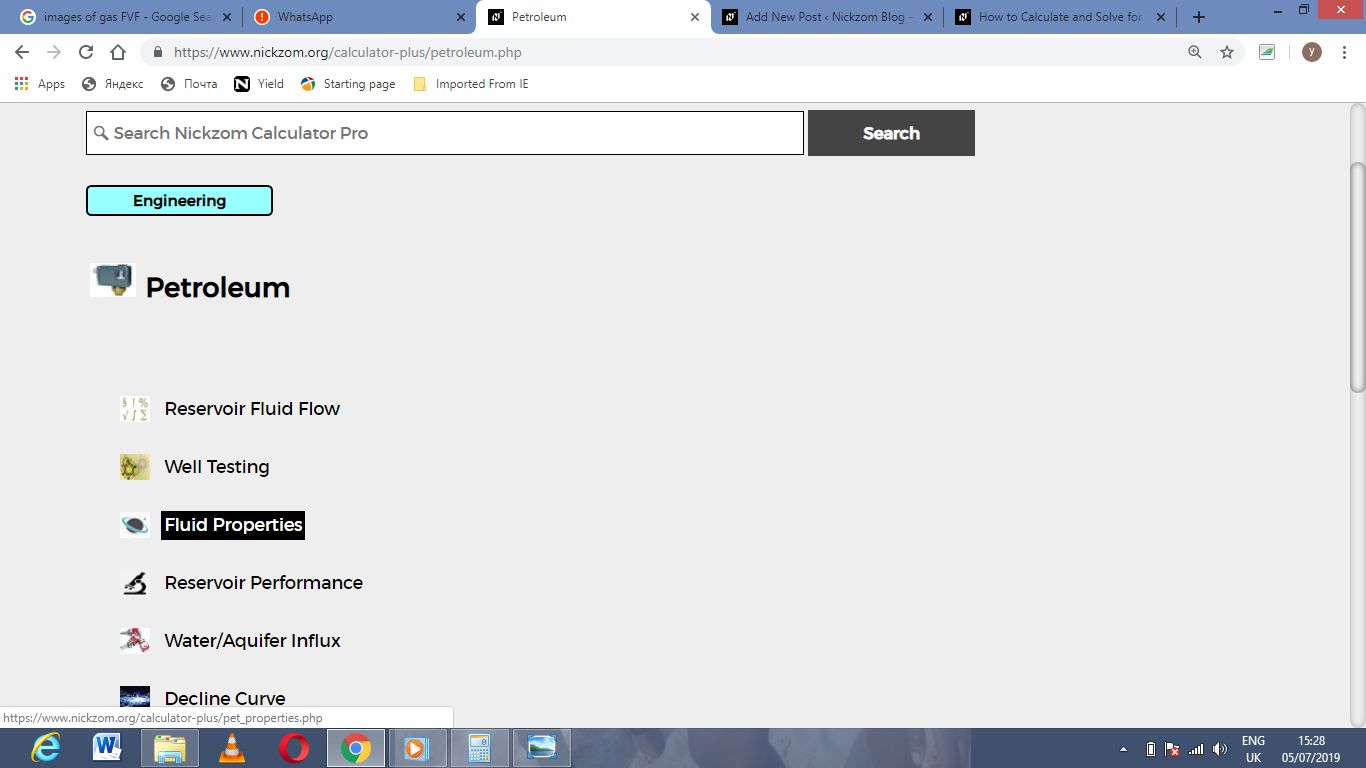Now, Click on Mean Bubble Point under Fluid Properties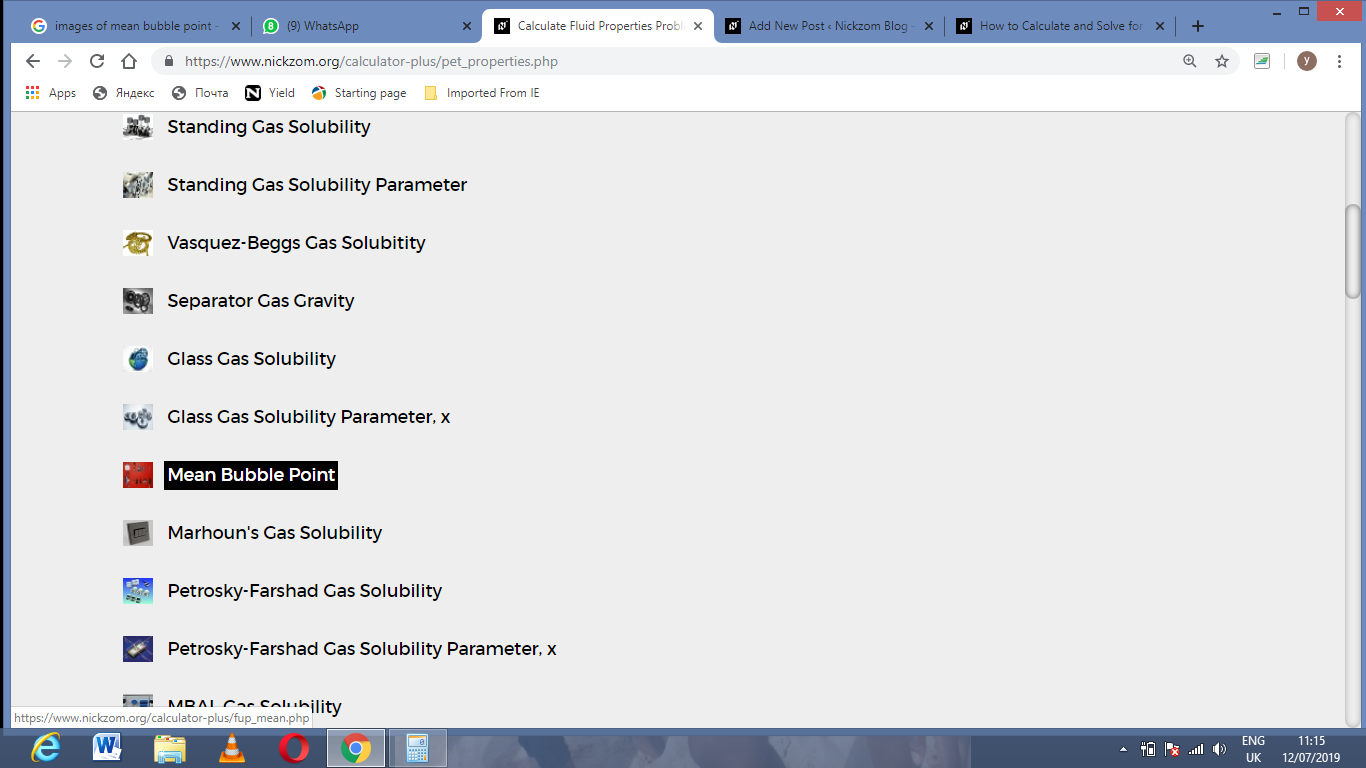The screenshot below displays the page or activity to enter your value, to get the answer for the mean bubble point according to the respective parameter which are the Glass gas solubility parameter (x).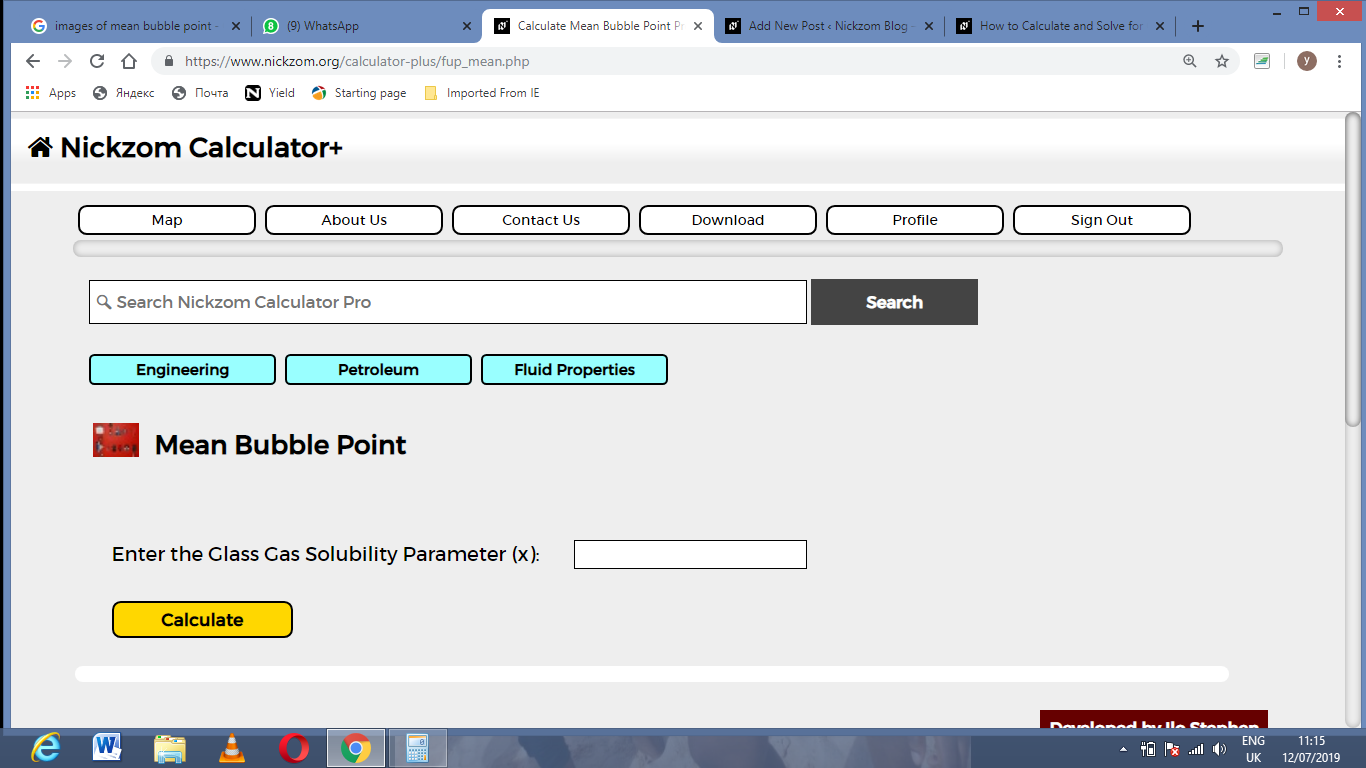Now, enter the value appropriately and accordingly for the parameter is required by the Glass gas solubility parameter (x) is 12.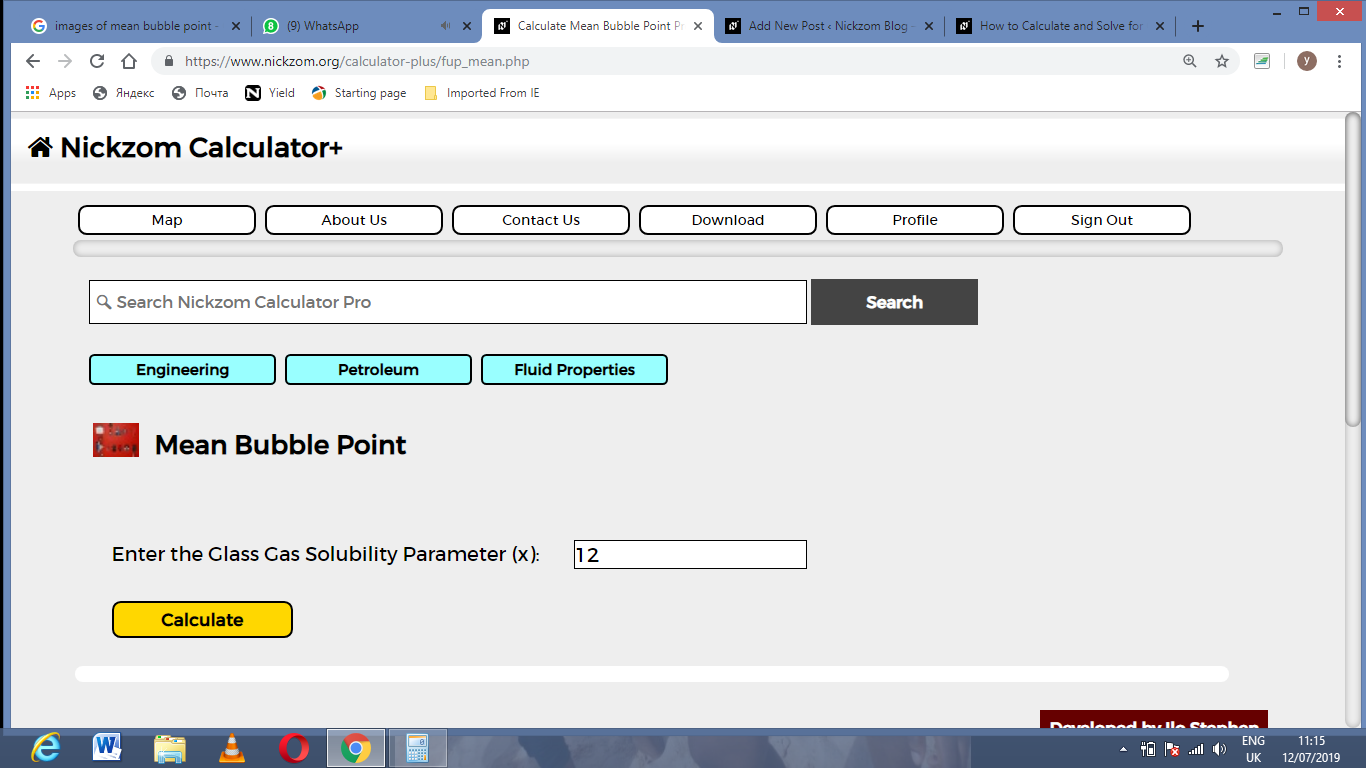Finally, Click on Calculate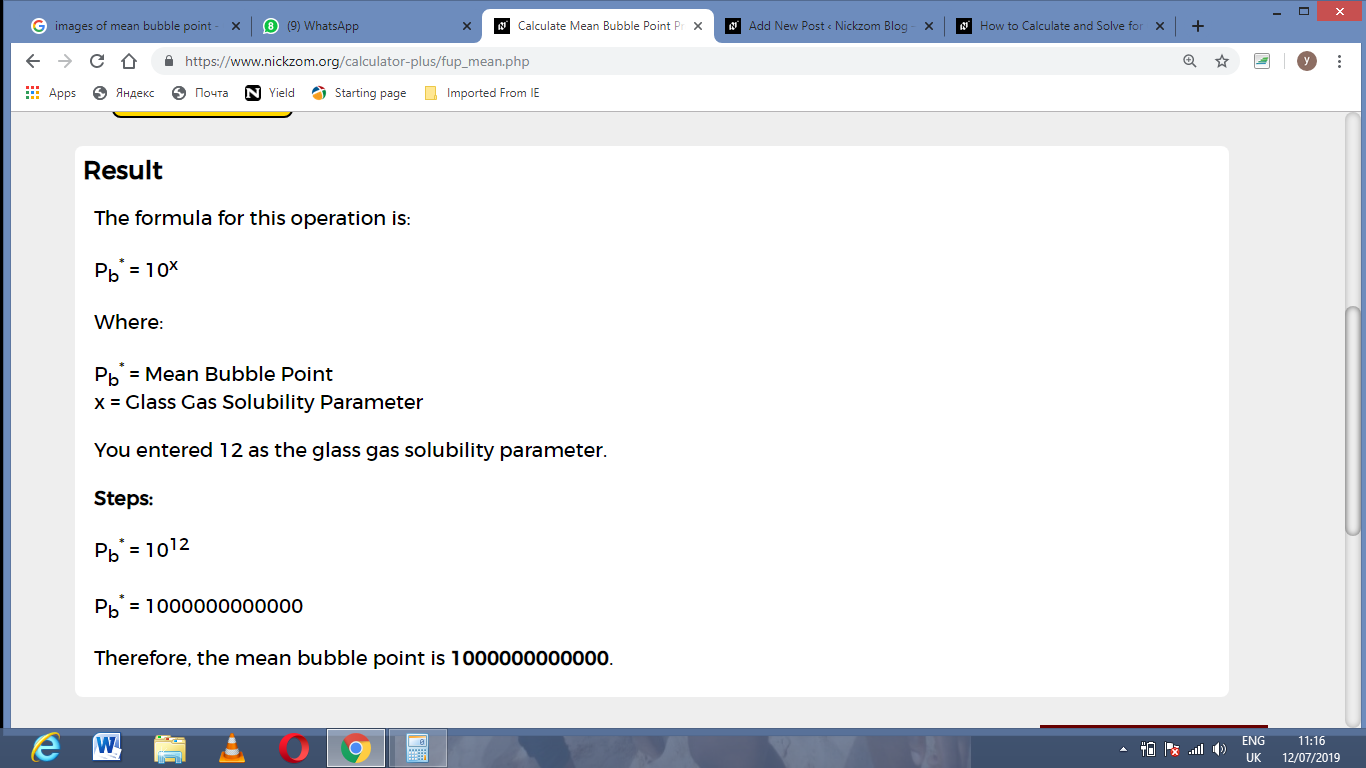As you can see from the screenshot above, Nickzom Calculator– The Calculator Encyclopedia solves for the mean bubble point and presents the formula, workings and steps too.